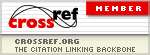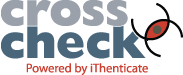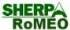### Optimization of “Deoxidation Alloying” Batching Scheme

Tingyu Ma, Tao Wang, Jingwen Huang, Lepan Wang, Xin Liu, Yingxia Liu, Hanjiang Dong, Dongsong Yan

#### Abstract

In this paper, a mathematical model was established to predict the deoxidation alloying and to optimize the type and quantity of input alloys. Firstly, the GCA method was used to obtain the main factors affecting the alloy yield of carbon and manganese based on the historical data. Secondly, the alloy yield was predicted by the stepwise MRA, the BP neural network and the regression SVM models, respectively. The conclusion is that the regression SVM model has the highest prediction accuracy and the maximum deviation between the test set prediction result and the real value was only 0.0682 and 0.0554. Thirdly, in order to reduce the manufacturer's production cost, the genetic algorithm was used to calculate the production cost mathematical programming model. Finally, sensitivity analysis was performed on the prediction model and the cost optimization model. The unit price of 20% of the alloy raw materials was increased by 20%, and the total cost change rate was 0.7155%, the lowest was -0.4297%, which proved that the mathematical model established presented strong robustness and could be certain reference value for the current production of iron and steel enterprises.

#### Full Text:

PDF

DOI: https://doi.org/10.11114/set.v7i1.4801

### Refbacks

• There are currently no refbacks.

Studies in Engineering and Technology   ISSN 2330-2038 (Print)   ISSN 2330-2046 (Online)